## Abstract

A mathematical study is presented to analyze the nonlinear, non-isothermal, magnetohydrodynamic (MHD) free convection boundary layer flow, heat and mass transfer of non-Newtonian Eyring–Powell fluid from a vertical surface in a non-Darcy, isotropic, homogenous porous medium, in the presence of Hall currents and ionslip currents. The governing nonlinear coupled partial differential equations for momentum conservation in the x, and z directions, heat and mass conservation, in the flow regime are transformed from an (xyz) coordinate system to a (ξη) coordinate system in terms of dimensionless x-direction velocity (f′) and z-direction velocity (G), dimensionless temperature and concentration functions (θ and ϕ) under appropriate boundary conditions. Both Darcian and Forchheimer porous impedances are incorporated in both momentum equations. Computations are also provided for the variation of the x and z direction shear stress components and also heat and mass transfer rates. It is observed that with increasing ɛ, primary velocity, secondary velocity, heat and mass transfer rates are decreased whereas, the temperature, concentration and skin friction are increased. An increasing δ is found to increase primary and secondary velocities, skin friction, heat and mass transfer rates. But the temperature and concentration decrease. Increasing βe and βi are seen to increase primary velocity, skin friction, heat and mass transfer rates whereas secondary velocity, temperature and concentration are decreased. Excellent correlation is achieved with a Nakamura tridiagonal finite difference scheme (''NTM''). The model finds applications in magnetic materials processing, MHD power generators and purification of crude oils.

## Keywords

Non-Newtonian Eyring–Powell model; Viscous dissipation; Non-Darcy model; Hall currents; Ionslip currents; Forchheimer parameter

## 1. Introduction

Analysis of non-Newtonian fluids has been the focus of several investigations during the past few decades because of its extensive engineering and industrial applications. Especially, flow and heat transfer of non-Newtonian fluids play central role in food engineering, petroleum production, power engineering and in polymer solutions and in polymer melt in the plastic processing industries. In nature there are non-Newtonian fluids with different characteristics and consequently various constitutive equations of non-Newtonian fluids have been presented. The use of such constitutive equations leads to more complicated and highly nonlinear systems. The analytic/numerical solutions of such equations present exciting challenges to the mathematicians, physicists, numerical simulists and modelers. Significant attention has been directed at mathematical and numerical simulation of non-Newtonian fluids. Recent investigations have implemented, respectively the Casson model , tangent hyperbolic model , Eyring–Powell fluid model  and Jeffreys viscoelastic model .

Heat transfer in the presence of strong magnetic fields is important in various branches of magnetohydrodynamic power generation, nanotechnological processing, nuclear energy systems exploiting liquid metals and blood flow control. Nadeem et al.  investigated the MHD stagnation point flow of Carreau fluid toward a permeable shrinking sheet: Dual solutions. Akbar et al.  examined the dual solutions in MHD stagnation-point flow of Prandtl fluid impinging on shrinking sheet. Nadeem et al.  investigated the MHD three-dimensional Casson fluid flow past a porous linearly stretching sheet. Radiation effects on MHD stagnation point flow of nanofluid toward a stretching surface with convective boundary condition were analyzed by Akbar et al. . Nadeem et al.  examined the MHD three-dimensional boundary layer flow of Casson nanofluid past a linearly stretching sheet with convective boundary condition. Akbar et al.  investigated the MHD boundary layer flow of tangent hyperbolic fluid toward a stretching sheet using fourth and fifth order Runge–Kutta–Fehlberg method. Hall currents and Ionslip effects become significant in strong magnetic fields and can considerably affect the current density in hydromagnetic heat transfer. Joule heating effects are also important and are caused by heating of the electrically-conducting fluid by the electrical current. As such considerable attention has been devoted to studying hydromagnetic convection flows with such effects. Hossain  reported on the effects of Hall currents on transient natural convection MHD boundary layer with suction at the wall using the Keller-Box numerical method. Raju and Rao  studied the cases of conducting and non-conducting walls for ionized hydromagnetic rotating heat transfer in a parallel plate channel with Hall currents. They showed that the temperature field is independent of partial pressure of electron gas for the case of non-conducting walls. Sawaya et al.  determined experimentally the Hall parameter for electrolytic solutions in a closed loop thermo symphonic magnetohydrodynamic flow system. A one dimensional theoretical model with the measured open circuit voltage was used to quantify Hall parameter. Bhargava and Takhar  studied computationally the influence of Hall currents on hydromagnetic heat transfer of a viscoelastic fluid in a channel. These studies did not consider however the presence of ion-slip currents. In weaker magnetic fields, the diffusion velocity of electrons and ions is different and usually ionslip effects are neglected. However in MHD generators and industrial materials processing where the electromagnetic body forces are large, the diffusion velocity of the ions cannot be neglected. When both electron and ion velocities are incorporated in the analysis, the ionslip phenomenon is present and Ohm’s law has to be modified accordingly. An excellent discussion of ionslip effects has been presented by Cramer and Pai . Soundalgekar et al.  were among the first researchers to consider ionslip effects in hydromagnetic Couette heat transfer in addition to Hall currents effects. They showed that for small magnetic field parameters or high ionslip and Hall current parameters, the flow can become unstable. Ram and Takhar  reported on the rotating natural convection MHD flow with Hall/ionslip current effects. Ram et al.  extended this study to consider the effects of oscillating wall temperature using a numerical method. Further studies of combined Hall and ionslip currents in magnetohydrodynamic heat transfer flows were provided by Takhar and Jha  and more recently by Anwar Beg et al. . Several studies of Joule electrical heating in MHD heat transfer flows have also appeared. Mansour and Gorla  studied the effects of Joule heating effects on transient free hydromagnetic convection in a micropolar fluid. Bég  studied the effects of Joule heating in MHD channel flow using a Navier–Stokes computational solver. Aissa and Mohammadein  more recently analyzed the effects of Joule heating and variable electric conductivity on micropolar stretching flow and heat transfer using a shooting numerical scheme. The combined effects of Hall current, magnetic induction and oblique magnetic field on MHD flow in a spinning channel with heat transfer were studied by Ghosh et al. . Other studies incorporating Joule heating have been communicated by Duwairi  and Bég et al.  who employed an electrothermal network simulation code.

In many industrial and geophysical flows viscous dissipation effects may also arise owing to internal friction in viscous fluids which can affect temperature fields. Many studies have been reported concerning viscous heating effects in both natural and forced convection heat transfer flows. These include the articles by Gebhart and Mollendorf  and Soundalgekar and Pop  which dwell on boundary layer heat transfer. In hydromagnetic heat transfer several studies have been reported concerning viscous heating effects. Javeri  studied hydromagnetic heat transfer in a channel with the collective effects of Hall current, ion slip, viscous dissipation and Joule heating. Takhar and Soundalgekar  presented numerical solutions for the effects of Eckert number (viscous heating parameter) on hydromagnetic natural convection boundary layer flow. Other non-magnetic studies of viscous heating effects include those by Turcotte et al. , Basu and Roy , Barletta  and Barletta and Rossi di Schio . These studies have all been restricted to purely fluid regimes. In numerous systems the medium may be a porous material. The porosity of materials is an intrinsic aspect of many chemical engineering and materials processing systems. Ceramics, batch reactors, purification systems and filtration systems all utilize porosity. Traditionally, the Darcian model has been employed to analyze most convection flows in porous media. Such a model however is generally only accurate at very low Reynolds numbers and cannot simulate the inertial effects experienced at higher Reynolds numbers. Engineers have therefore extended the Darcian model to incorporate second order drag force effects generally with the Forchheimer-extended Darcian model, which is easily implemented in boundary layer heat transfer analysis. Excellent studies of Darcy–Forchheimer convection in porous media have been presented by for example, Chen and Ho  and also Manole and Lage .

An interesting non-Newtonian model developed for chemical engineering systems is the Eyring–Powell fluid model. This rheological model has certain advantages over the other non-Newtonian formulations, including simplicity, ease of computation and physical robustness. Furthermore, it is deduced from kinetic theory of liquids rather than the empirical relation. Additionally, it correctly reduces to Newtonian behavior for low and high shear rates . Several communications utilizing the Eyring–Powell fluid model have been presented in the scientific literature. Zueco and Bég  numerically studied the pulsatile flow of Eyring–Powell model using the network electro-thermal solver code, PSPICE. Islam et al.  derived homotopy perturbation solutions for slider bearings lubricated with Eyring–Powell fluids. Patel and Timol  numerically examined the flow of Eyring–Powell fluids from a two-dimensional wedge. Further investigations implementing the Eyring–Powell model in transport phenomena include Ramachandra Prasad et al.  for vertical porous plate flows, Akbar et al.  for peristaltic thermal convection flows of reactive biofluids, Hayat et al.  for Sakiadis flows and Abdul Gaffar et al.  for wedge flows. The Eyring–Powell model was also deployed by Yürüsoy  to investigate bearing tribological flows, by Ai and Vafai  to simulate Stokesian impulsively-started plate flows and also by Adesanya and Gbadeyan  for channel flows. Recent work on Eyring–Powell fluid includes Abdul Gaffar et al. .

The above studies however did not consider the mass transfer and the collective effects of Joule heating, Hall/ionslip currents in porous media transport phenomena. The vast majority of simulations employ a Darcian model , valid for viscous-dominated flows. In this paper we shall therefore, consider the composite effects of Joule heating, Hall and ionslip currents, and also viscous frictional heating on two-dimensional natural MHD convection of Eyring–Powell Fluid in a Darcy-Forchheimer porous medium from a vertical plate. Such a study has thus far not appeared in the literature and constitutes a useful extension to the current body of work on non-linear magneto-convective transport phenomena in porous media.

## 2. Non-newtonian constitutive Eyring–Powell fluid model

In the present study a subclass of non-Newtonian fluids known as Eyring–Powell fluid is employed owing to its simplicity. The Cauchy stress tensor, in as Eyring–Powell fluid  takes the following form:

 ${\tau }_{ij}=\mu {\frac {\partial u_{i}}{\partial x_{j}}}+$${\frac {1}{\beta }}{sinh}^{-1}\left({\frac {1}{C}}{\frac {\partial u_{i}}{\partial x_{j}}}\right)$
(1)

where μ   is dynamic viscosity, and ${\textstyle \beta }$ and C are the rheological fluid parameters of the Eyring–Powell fluid model. Consider the second-order approximation of the sinh−1 function as follows:

 ${sinh}^{-1}\left({\frac {1}{C}}{\frac {\partial u_{i}}{\partial x_{j}}}\right)\cong {\frac {1}{C}}{\frac {\partial u_{i}}{\partial x_{j}}}-$${\frac {1}{6}}{\left({\frac {1}{C}}{\frac {\partial u_{i}}{\partial x_{j}}}\right)}^{3}{\mbox{,}}\quad \left|{\frac {1}{C}}{\frac {\partial u_{i}}{\partial x_{j}}}\right|\ll 1$
(2)

The introduction of the appropriate terms into the flow model is considered next. The resulting boundary value problem is found to be well-posed and permits an excellent mechanism for the assessment of rheological characteristics on the flow behavior.

## 3. Mathematical model

We consider the steady-state hydromagnetic natural convection flow of a viscous, incompressible, partially-ionized, electrically-conducting flow of Eyring–Powell fluid adjacent to a non-isothermal, vertical surface in an (xyz) coordinate system embedded in a non-Darcy saturated porous medium. The plate surface is in the xz plane. The z-axis coincides with the leading edge of the plate. A strong magnetic field acts parallel to the y-axis. The physical model is illustrated in Fig. 1. The magnetic Reynolds number is small for the partially-ionized fluid so that magnetic induction effects can be ignored. However, relative motion of the particles in the fluid can occur and the electron-atom collision frequency is assumed to be high enough for Hall and ionslip currents to be significant. As such, an electric current density, J, is required to represent the relative motion of charged particles. Considering only the electromagnetic forces on these particles, we can utilize the generalized Ohm law. With a magnetic field, B, applied normal to the electrical field E, an electromagnetic force is generated normal to both E and B in the z direction. Such a force causes charged particles to migrate perpendicularly to both E and B. Consequently, a component of electrical current density exists perpendicular to both E and B, and this constitutes the Hall current. For a strong magnetic field B the diffusion velocity of the ions will be significant, constituting the ionslip effect. From the equation of conservation of electrical charge:

 $\nabla \cdot J=0$
(4)

where J = (JxJyJz). Since the plate is not composed of electrically-conducting material, electrical charge at the surface of the plate is constant and zero i.e. Jy → 0. Consequently we can assume that Jy = 0 throughout the fluid-saturated porous medium. The magnetic field acts only in the y-direction with a component, Bo. Both the vertical surface and the Eyring–Powell fluid are maintained initially at the same temperature and concentration. Instantaneously, they are raised to a temperature Tw (>T) and concentration Cw (>C), the ambient temperature and concentration of the fluid which remain unchanged. We implement a Darcy–Forchheimer model which is a second order relationship defining the pressure gradient as follows:

 $\nabla p=-aU+{bU}^{2}$
(5)

where U denotes velocity, ∇p is pressure gradient, a and b are constants defined by a = μ/K and b is a function of the geometry of porous medium i.e. b = ρ/K1 is the Forchheimer form-drag parameter for quadratic effects, μ is the dynamic viscosity of the partially-ionized fluid, K is permeability [hydraulic conductivity] of the porous medium and K1 is the inertial permeability. We assume that the density of the partially-ionized fluid can be taken as constant i.e. the flow is incompressible. In addition, we implement the Boussinesq approximation which implies that all thermodynamic quantities of the fluid-saturated medium are constant, except for the buoyancy term, which is retained in the x-direction momentum conservation equations. We have assumed that the porous medium is homogenous and isotropic so that only a single permeability is needed to simulate hydraulic conductivity. Under these physical conditions, with viscous and Joule heating effects incorporated, the flow regime in an [xyz] coordinate system can be represented by the following boundary-layer equations:

 ${\frac {\partial u}{\partial x}}+{\frac {\partial v}{\partial y}}=$$0$
(6)

 $u{\frac {\partial u}{\partial x}}+v{\frac {\partial u}{\partial y}}=$$\left[\nu +{\frac {1}{\rho \beta C_{1}}}\right]{\frac {{\partial }^{2}u}{\partial y^{2}}}-$${\frac {1}{2\rho \beta C_{1}^{3}}}{\left({\frac {\partial u}{\partial y}}\right)}^{2}{\frac {{\partial }^{2}u}{\partial y^{2}}}+$$g{\beta }_{1}[T-T_{\infty }]+g{\beta }_{1}^{_{\ast }}[C-$$C_{\infty }]-\nu {\frac {u}{K}}-b{\frac {u^{2}}{K}}-{\frac {\sigma B_{o}^{2}}{\rho ({\alpha }_{e}^{2}+{\beta }_{e}^{2})}}({\alpha }_{e}u+$${\beta }_{e}w)$
(7)

 $u{\frac {\partial w}{\partial x}}+v{\frac {\partial w}{\partial y}}=$$\left[\nu +{\frac {1}{\rho \beta C_{1}}}\right]{\frac {{\partial }^{2}w}{\partial y^{2}}}-$${\frac {1}{2\rho \beta C_{1}^{3}}}{\left({\frac {\partial w}{\partial y}}\right)}^{2}{\frac {{\partial }^{2}w}{\partial y^{2}}}-$$\nu {\frac {w}{K}}-b{\frac {w^{2}}{K}}+{\frac {\sigma B_{o}^{2}}{\rho ({\alpha }_{e}^{2}+{\beta }_{e}^{2})}}({\beta }_{e}u-$${\alpha }_{e}w)$
(8)

 $u{\frac {\partial T}{\partial x}}+v{\frac {\partial T}{\partial y}}=$${\frac {k}{\rho c_{p}}}{\frac {{\partial }^{2}T}{\partial y^{2}}}+$${\frac {\nu }{c_{p}}}\left[{\left({\frac {\partial u}{\partial y}}\right)}^{2}+\right.$$\left.{\left({\frac {\partial w}{\partial y}}\right)}^{2}\right]+$${\frac {\sigma B_{o}^{2}}{\rho c_{p}({\alpha }_{e}^{2}+{\beta }_{e}^{2})}}(u^{2}+$$w^{2})$
(9)

 $u{\frac {\partial C}{\partial x}}+v{\frac {\partial C}{\partial y}}=$$D_{m}{\frac {{\partial }^{2}C}{\partial y^{2}}}$
(10)

where u, v, w are velocity components in the x, y, z directions, T is the fluid temperature, C is the fluid concentration, ν is kinematic viscosity of the partially-ionized fluid, g is acceleration due to gravity, K is the thermal diffusivity, b is the inertial drag coefficient, C1 is the fluid parameter, β is the fluid parameter, β1 is the coefficient of thermal expansion, β1 is the coefficient of expansion with concentration, ρ is density of the electrically-conducting fluid, k is thermal conductivity of the fluid-saturated porous medium, Dm, is the mass (species) diffusivity, cp is specific heat capacity of the fluid under isobaric conditions, σ is the fluid electrical conductivity (=e2tene/me), e denotes the electron charge, te is the electron collision time, ne is the electron number density, me is the electron mass, αe = 1 + βiβe  , ${\textstyle {\beta }_{i}={\frac {{en}_{e}B_{o}}{\left[\left(1+{\frac {n_{e}}{n_{a}}}\right)K_{ai}\right]}}}$ is the ion-slip parameter, βe = ωete is the Hall current parameter, ωe is the electron frequency (=eBo/me), na is the neutral particle number density and Kai is the friction coefficient between the ions and neutral particles in the flow. The Eyring–Powell fluid model introduces a mixed derivative (second order, first degree) into the momentum boundary layer Eq. (7). In Eq. (7) the fifth and sixth terms on the right hand side are the x-direction Darcian drag force and the x-direction Forchheimer quadratic drag. Similar terms are incorporated for the z-direction components of these forces in Eq. (8). We note that the viscous heating term in (9) comprises the square of two velocity gradients ${\textstyle \left[{\left({\frac {\partial u}{\partial y}}\right)}^{2}+\right.}$$\left.{\left({\frac {\partial v}{\partial y}}\right)}^{2}\right]$ which is a realistic approximation, supported by the seminal study of Gebhart and Mollendorf . The corresponding initial conditions and boundary conditions for the flow regime are prescribed as follows, which include the conventional no-slip boundary condition at the plate surface:

 ${\mbox{At}}\quad y=0:\quad u=v=0{\mbox{,}}\quad w=0{\mbox{,}}\quad T=$$T_{w}{\mbox{,}}\quad C=C_{w}$
(11a)

 ${\mbox{As}}\quad y\rightarrow \infty :\quad u\rightarrow 0{\mbox{,}}\quad w\rightarrow 0{\mbox{,}}\quad T\rightarrow T_{\infty }{\mbox{,}}\quad C\rightarrow C_{\infty }$
(11b)

Here T and C are free stream temperature and concentration respectively.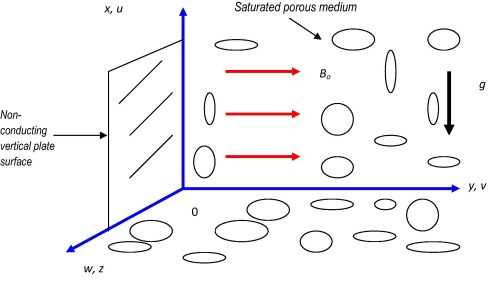Figure 1. Physical model and coordinate system.

To transform the boundary value problem to a dimensionless one, i.e. coupled non-linear partial differential Eqs. (6), (7), (8), (9) and (10) under conditions (11a) and (11b), we introduce a stream function Ψ   defined by the Cauchy–Riemann equations, ${\textstyle u={\frac {\partial \psi }{\partial y}}}$ and ${\textstyle v=-{\frac {\partial \psi }{\partial x}}}$, and therefore, the mass conservation Eq. (6) is automatically satisfied. Furthermore, the following dimensionless variables are introduced into Eqs. (6), (7), (8), (9) and (10) and (11a) and (11b):

 ${\begin{array}{rl}&\eta ={\frac {C_{1}y}{x^{1/4}}}{\mbox{,}}\quad \xi ={\frac {x^{1/2}}{L^{1/2}}}{\mbox{,}}\quad \Psi =4\nu C_{1}x^{3/4}f(\xi {\mbox{,}}\eta ){\mbox{,}}\quad w=4\nu C_{1}^{2}x^{1/2}G(\xi {\mbox{,}}\eta ){\mbox{,}}\quad \epsilon ={\frac {1}{\mu \beta C_{1}}}{\mbox{,}}\quad \delta =8{\nu }^{2}C_{1}^{3}L^{1/2}\\&\theta ={\frac {T-T_{\infty }}{T_{w}-T_{\infty }}}{\mbox{,}}\quad \phi ={\frac {C-C_{\infty }}{C_{w}-C_{\infty }}}{\mbox{,}}\quad Pr={\frac {\mu c_{p}}{k}}{\mbox{,}}\quad {Gr}_{x}={\frac {g\beta x^{3}[T_{w}-T_{\infty }]}{{\nu }^{2}}}{\mbox{,}}\quad Ec={\frac {4L{\nu }^{2}C_{1}^{4}}{c_{p}[T_{w}-T_{\infty }]}}\\&N_{m}={\frac {\sigma B_{o}^{2}L^{1/2}}{2\rho \nu C_{1}^{2}}}{\mbox{,}}\quad Da={\frac {K}{L^{2}}}{\mbox{,}}\quad Fs={\frac {b}{L}}{\mbox{,}}\quad Sc={\frac {\nu }{D_{m}}}{\mbox{,}}\quad C_{1}={\left\{{\frac {g\beta [T_{w}-T_{\infty }]}{4{\nu }^{2}}}\right\}}^{1/4}\end{array}}$
(12)

where L denotes a characteristic length, F is dimensionless stream function, G is dimensionless lateral velocity, θ is dimensionless temperature, ϕ is dimensionless concentration, ξ is dimensionless tangential coordinate, η is the dimensionless normal coordinate, Ψ is dimensionless stream function, Nm is the magnetic body force parameter, Pr is Prandtl number, Grx is local Grashof number, Ec is Eckert (viscous heating) number, Da is Darcy number, ε is the Eyring–Powell fluid parameter, δ is the local non-Newtonian fluid parameter, the local non-Newtonian parameter based on length scale, and Fs is Forchheimer (inertial) parameter. The resulting momentum, energy and concentration boundary layer equations take the following form:

 $(1+\epsilon )f^{'''}+3{ff}^{''}-2f^{'}{\quad }^{2}-$$\epsilon \delta \quad \xi {\left(f^{''}\right)}^{2}f^{'''}+$$(\theta +N\phi )-{\frac {2}{Da\quad {Gr}_{x}^{1/2}}}{\xi }^{4}f^{'}-$${\frac {4Fs}{Da}}{\xi }^{2}f^{'}{\quad }^{2}\quad -{\frac {2N_{m}}{\left[{\alpha }_{e}^{2}+{\beta }_{e}^{2}\right]}}\xi [{\alpha }_{e}f^{'}+$${\beta }_{e}G]=2\xi \left[f^{'}{\frac {\partial f^{'}}{\partial \xi }}-\right.$$\left.f^{''}{\frac {\partial f}{\partial \xi }}\right]$
(13)

 $(1+\epsilon )G^{''}+3f\quad G^{'}-2{Gf}^{'}-$$\epsilon \delta \quad \xi {\left(G^{'}\right)}^{2}G^{''}-$${\frac {2}{Da\quad {Gr}_{x}^{1/2}}}{\xi }^{4}G-{\frac {4Fs}{Da}}{\xi }^{2}G^{2}\quad -$${\frac {2N_{m}}{\left[{\alpha }_{e}^{2}+{\beta }_{e}^{2}\right]}}\xi [{\alpha }_{e}f^{'}+$${\beta }_{e}G]=2\xi \left[f^{'}{\frac {\partial f^{'}}{\partial \xi }}-\right.$$\left.f^{''}{\frac {\partial f}{\partial \xi }}\right]$
(14)

 ${\frac {1}{Pr}}{\theta }^{''}+3f{\theta }^{'}+4{\xi }^{2}Ec[f^{''}{\quad }^{2}+$$G^{'}{\quad }^{2}]+{\frac {2N_{m}\quad Ec}{\left[{\alpha }_{e}^{2}+{\beta }_{e}^{2}\right]}}{\xi }^{3}[f^{{'}2}+$$G^{2}]=2\xi \left[f{\frac {\partial \theta }{\partial \xi }}-\right.$$\left.{\theta }^{'}{\frac {\partial f}{\partial \xi }}\right]$
(15)

 ${\frac {1}{Sc}}{\phi }^{''}+3f{\phi }^{'}=2\xi \left[f^{'}{\frac {\partial \phi }{\partial \xi }}-\right.$$\left.{\phi }^{'}{\frac {\partial f}{\partial \xi }}\right]$
(16)

The corresponding transformed non-dimensional boundary conditions take the following form:

 ${\mbox{At}}\quad \quad \eta =0{\mbox{,}}\quad f=0{\mbox{,}}\quad u=$$0{\mbox{,}}\quad G=0{\mbox{,}}\quad \theta =0{\mbox{,}}\quad \phi =$$0$
(17a)

 ${\mbox{As}}\quad \quad \eta \rightarrow \infty {\mbox{,}}\quad f^{'}\rightarrow 0{\mbox{,}}\quad G\rightarrow 0{\mbox{,}}\quad \theta \rightarrow 0{\mbox{,}}\quad \phi \rightarrow 0$
(17b)

Here primes denote the differentiation with respect to η.

## 4. Engineering parameters

For practical design purposes in MHD energy systems a number of engineering parameters provide important descriptions of the wall transport processes. The local heat flux, qw(x), local heat transfer coefficient, h(x) at the wall and the heat transfer rate, Nux are defined as follows:

 $q_{w}(x)=-k{\frac {\partial T}{\partial y}}_{y=0}=-k[T_{w}-$$T_{\infty }]{\frac {C_{1}}{x^{1/4}}}{\frac {\partial \theta (\xi {\mbox{,}}0)}{\partial \eta }}$
(18)
 $h(x)={\frac {q_{w}(x)}{\left[T_{w}-T_{\infty }\right]}}=$$-k{\frac {C_{1}}{x^{1/4}}}{\frac {\partial \theta (\xi {\mbox{,}}0)}{\partial \eta }}$
(19)
 ${Nu}_{x}={\frac {xh(x)}{k}}=-{\frac {1}{\sqrt {2}}}{Gr}_{x}^{1/4}{\frac {\partial \theta (\xi {\mbox{,}}0)}{\partial \eta }}$
(20)

The local shear stress components in the x and z-directions respectively, namely, τwx and τwz, may be defined using the following relations:

 ${\tau }_{wx}={\sqrt {2}}\quad \mu \nu {\frac {{Gr}_{x}^{3/4}}{x^{2}}}\left[(1+\right.$$\left.\epsilon ){\frac {{\partial }^{2}f(\xi {\mbox{,}}0)}{\partial {\eta }^{2}}}-\right.$$\left.{\frac {\epsilon \delta }{3}}\xi {\left({\frac {{\partial }^{2}f(\xi {\mbox{,}}0)}{\partial {\eta }^{2}}}\right)}^{3}\right]$
(21a)
 ${\tau }_{wz}={\sqrt {2}}\quad \mu \nu {\frac {{Gr}_{x}^{3/4}}{x^{2}}}\left[(1+\right.$$\left.\epsilon ){\frac {\partial G(\xi {\mbox{,}}0)}{\partial \eta }}-\right.$$\left.{\frac {\epsilon \delta }{3}}\xi {\left({\frac {\partial G(\xi {\mbox{,}}0)}{\partial \eta }}\right)}^{3}\right]$
(21b)

The dimensionless skin friction coefficients in x and z-directions respectively, namely, Cfx and Cgz are defined as,

 $C_{fx}=(1+\epsilon ){\frac {{\partial }^{2}f(\xi {\mbox{,}}0)}{\partial {\eta }^{2}}}-$${\frac {\epsilon \delta }{3}}\xi {\left({\frac {{\partial }^{2}f(\xi {\mbox{,}}0)}{\partial {\eta }^{2}}}\right)}^{3}$
(22a)
 $C_{gz}=(1+\epsilon ){\frac {\partial G(\xi {\mbox{,}}0)}{\partial \eta }}-$${\frac {\epsilon \delta }{3}}\xi {\left({\frac {\partial G(\xi {\mbox{,}}0)}{\partial \eta }}\right)}^{3}$
(22b)

The mass transfer rate, Shx is defined as,

 ${Sh}_{x}=-{\frac {1}{\sqrt {2}}}{Gr}_{x}^{1/4}{\frac {\partial \phi (\xi {\mbox{,}}0)}{\partial \eta }}$
(23)

To provide a benchmark for numerical solutions, a number of special cases of the general flow model can be retrieved from Eqs. (13), (14), (15) and (16) with conditions (17a) and (17b). With Fs → 0, inertial effects disappear and only a bulk porous matrix resistance (Darcian) acts on the partially-ionized fluid in the porous medium. With Da → ∞, the regime permeability becomes infinite and the porous fibers vanish in the limit. The model contracts to purely fluid hydromagnetic convection flow. For the case of Ec → 0, both viscous heating and Joule electric current heating terms vanish in the energy conservation equation. Finally, with absence of magnetic field effects, Nm → 0, which also negates Hall current and ionslip effects in the momentum equations.

## 5. Keller Box computational solutions

The Keller-Box implicit difference method (KBM) is utilized to solve the nonlinear boundary value problem defined by Eqs. (13), (14), (15) and (16) with boundary conditions (17a) and (17b). Although other powerful numerical methods have been developed in fluid mechanics including differential transform quadrature  and MAPLE shooting methods , for parabolic problems (of which boundary layer flows are an excellent example), Keller’s box technique  remains extremely popular since it easily solves two-coordinate problems (partial differential families of equations). Although Keller’s box scheme was developed over four decades ago, it has witnessed a recent re-surge in implementation. Recent areas in which this scheme has been employed include Jeffrey’s fluid  and , tangent hyperbolic fluid , magnetohydrodynamics , wavy surface convection flows , nanofluids  and drainage sheet flows . The Keller-Box discretization is fully coupled at each step which reflects the physics of parabolic systems – which are also fully coupled. Discrete calculus associated with the Keller-Box scheme has also been shown to be fundamentally different from all other mimetic (physics capturing) numerical methods, as elaborated by Keller . The Keller Box Scheme comprises four stages:

• Decomposition of the Nth order partial differential equation system to N first order equations.
• Finite Difference Discretization.
• Quasilinearization of Non-Linear Keller Algebraic Equations.
• Block-tridiagonal elimination solution of the Linearized Keller Algebraic Equations.

## 6. Keller box computational results and discussion

Comprehensive solutions have been obtained and are presented in Table 1, Table 2, Table 3 and Table 4 and Figure 2a, Figure 2b, Figure 2c, Figure 2d, Figure 3a, Figure 3b, Figure 3c, Figure 3d, Figure 4a, Figure 4b, Figure 4c, Figure 4d, Figure 5a, Figure 5b, Figure 5c, Figure 5d, Figure 6a, Figure 6b, Figure 6c, Figure 6d, Figure 7a, Figure 7b, Figure 7c, Figure 7d, Figure 8a, Figure 8b, Figure 8c, Figure 8d, Figure 9a, Figure 9b, Figure 9c, Figure 10a, Figure 10b, Figure 10c, Figure 11a, Figure 11b, Figure 11c, Figure 12a, Figure 12b, Figure 12c, Figure 13a, Figure 13b, Figure 13c, Figure 14a, Figure 14b, Figure 14c, Figure 15a, Figure 15b and Figure 15c. The numerical problem comprises two independent variables (ξη), four dependent fluid dynamic variables (fGθϕ) and different thermo-physical and body force control parameters, namely, ε, δ, βe, βi, Nm, Pr, Da, Fs, Gr, Ec. The following default parameter values i.e. ε = 0.1, δ = 0.3, βe = 0.5, βi = 0.4, Nm = 0.5, Pr = 7.0, Gr = 1.0, Ec = Da = Fs = 0.1 are prescribed (unless otherwise stated). Validation with Nakamura’s tridiagonal finite difference method (NTM)  is provided in the next section with Table 5 and Table 6.

Table 1. Values of Cfx, Nux, and Shx for different ε, βe, βi and ξ (δ = 0.3, Nm = 0.5, Sc = 0.6, Gr = 1.0, Fs = Ec = Da = 0.1, N = 0.5, Pr = 7.0).
ε βe βi ξ = 1.0 ξ = 2.0 ξ = 3.0
Cfx Nux Shx Cfx Nux Shx Cfx Nux Shx
0.0 0.5 0.4 0.2752 0.5246 0.1713 0.0794 0.1140 0.1965 0.0363 0.2002 0.2170
0.18 0.2873 0.5216 0.1705 0.0855 0.1132 0.1950 0.0392 0.1935 0.2099
0.35 0.3007 0.5197 0.1683 0.0914 0.1127 0.1933 0.0420 0.1874 0.2042
0.52 0.3164 0.5193 0.1661 0.0972 0.1114 0.1931 0.0446 0.1850 0.2020
0.74 0.3333 0.5189 0.1654 0.1035 0.1105 0.1928 0.0477 0.1842 0.2014
1.0 0.3543 0.5162 0.1637 0.1105 0.1087 0.1922 0.0508 0.1822 0.1997
0.1 0.1 0.4 0.2806 0.5171 0.1658 0.0825 0.1088 0.1924 0.0375 0.1772 0.2003
0.57 0.2818 0.5200 0.1645 0.0826 0.1090 0.1921 0.0375 0.1777 0.1994
1.0 0.2826 0.5220 0.1635 0.0826 0.1091 0.1918 0.0376 0.1783 0.1988
2.0 0.2836 0.5251 0.1623 0.0826 0.1095 0.1916 0.0377 0.1799 0.1983
3.0 0.2840 0.5258 0.1618 0.0827 0.1098 0.1915 0.0378 0.1815 0.1980
5.0 0.2843 0.5423 0.1614 0.0828 0.1102 0.1914 0.0380 0.1839 0.1979
0.1 0.5 0.0 0.2812 0.5185 0.1617 0.0826 0.1089 0.1915 0.0375 0.1776 0.1980
1.0 0.2821 0.5208 0.1620 0.0826 0.1090 0.1916 0.0375 0.1780 0.1982
3.0 0.2830 0.5230 0.1624 0.0826 0.1092 0.1916 0.0376 0.1786 0.1984
5.0 0.2834 0.5241 0.1629 0.0826 0.1093 0.1918 0.0376 0.1793 0.1987
8.0 0.2838 0.5248 0.1641 0.0827 0.1097 0.19120 0.0378 0.1810 0.1992
12.0 0.2840 0.5254 0.1652 0.0827 0.1099 0.1922 0.0378 0.1825 0.1997

Table 2. Values of Cfx, Nux, and Shx for different Sc, Pr and ξ (βe = 0.5, βi = 0.4, ε = 0.1, δ = 0.3, Gr = 1.0, Ec = Da = Fs = 0.1, Nm = N = 0.5).
Sc Pr ξ = 1.0 ξ = 2.0 ξ = 3.0
Cfx Nux Shx Cfx Nux Shx Cfx Nux Shx
0.25 7.0 0.2614 0.5244 0.1617 0.0758 0.1115 0.1727 0.0346 0.1962 0.1989
0.75 0.2614 0.5235 0.1645 0.0757 0.1110 0.1852 0.0346 0.1937 0.2015
0.98 0.2612 0.5202 0.1751 0.0756 0.1106 0.1884 0.0344 0.1893 0.2061
1.25 0.2612 0.5185 0.1849 0.0755 0.1099 0.1899 0.0344 0.1825 0.2101
2.0 0.2607 0.5157 0.2202 0.0755 0.1096 0.1971 0.0345 0.1816 0.2124
0.6 0.71 0.2753 0.1544 0.1629 0.0755 0.1188 0.1929 0.0345 0.1936 0.2037
1.0 0.2744 0.1777 0.1597 0.0754 0.1483 0.1928 0.0344 0.1978 0.2020
2.0 0.2712 0.2610 0.1572 0.0753 0.1701 0.1927 0.0344 0.1987 0.2014
3.0 0.2685 0.3294 0.1527 0.0753 0.1874 0.1926 0.0344 0.1994 0.1996
5.0 0.2645 0.4359 0.1501 0.0753 0.1912 0.1925 0.0343 0.1996 0.1987

Table 3. Values of Cfx, Nux, and Shx for different δ, Nm, Fs and ξ (ε = 0.1, βe = 0.5, βi = 0.4, Gr = 1.0, Ec = Da = 0.1 = Fs, N = 0.5, Sc = 0.6, Pr = 7.0).
δ Nm Fs ξ = 1.0 ξ = 2.0 ξ = 3.0
Cfx Nux Shx Cfx Nux Shx Cfx Nux Shx
0 0.5 0.1 0.2816 0.5111 0.1632 0.0802 0.0992 0.1886 0.0349 0.1573 0.1913
5 0.2823 0.5130 0.1635 0.0802 0.0985 0.1888 0.0349 0.1580 0.1908
10 0.2839 0.5142 0.1641 0.0808 0.1004 0.1894 0.0357 0.1619 0.1920
15 0.2858 0.5178 0.1628 0.0815 0.1007 0.1896 0.0364 0.1676 0.1930
20 0.2885 0.5197 0.1647 0.0824 0.1071 0.1933 0.0369 0.1872 0.2039
30 0.2970 0.5220 0.1652 0.0829 0.1096 0.1962 0.0380 0.2023 0.2183
0.3 0.01 0.1 0.2846 0.5265 0.1695 0.0831 0.1117 0.1934 0.0384 0.1908 0.2029
0.25 0.2831 0.5231 0.1682 0.0830 0.1111 0.1930 0.0381 0.1880 0.2018
0.75 0.2801 0.5162 0.1647 0.0826 0.1098 0.1921 0.0378 0.1818 0.1995
1.0 0.2787 0.5128 0.1628 0.0825 0.1092 0.1917 0.0376 0.1791 0.1986
1.2 0.2775 0.5102 0.1610 0.0823 0.1087 0.1914 0.0375 0.1767 0.1977
0.3 0.6 0.01 0.2813 0.5208 0.1700 0.0828 0.1072 0.1929 0.0379 0.1860 0.2018
0.25 0.2806 0.5196 0.1691 0.0828 0.1077 0.1928 0.0379 0.1858 0.2012
0.35 0.2800 0.5164 0.1676 0.0828 0.1086 0.1926 0.0379 0.1850 0.2022
0.5 0.2791 0.5146 0.1665 0.0828 0.1083 0.1925 0.0378 0.1844 0.2021
0.6 0.2785 0.5134 0.1635 0.0828 0.1082 0.1923 0.0378 0.1839 0.2010

Table 4. Values of Cfx, Nux, and Shx for different Da, N and ξ (βe = 0.5, βi = 0.4, ε = 0.1, δ = 0.3, Gr = 1.0, Ec = Fs = 0.1, Nm = 0.5, Sc = 0.6, Pr = 7.0).
Da N ξ = 1.0 ξ = 2.0 ξ = 3.0
Cfx Nux Shx Cfx Nux Shx Cfx Nux Shx
0.05 0.5 0.1939 0.3545 0.1640 0.0536 0.1098 0.1903 0.0245 0.1684 0.1892
0.06 0.2105 0.3927 0.1647 0.0587 0.1123 0.1921 0.0268 0.1744 0.1995
0.08 0.2383 0.4658 0.1647 0.0677 0.1146 0.1929 0.0309 0.1818 0.1971
0.1 0.2614 0.5196 0.1653 0.0755 0.1209 0.1957 0.0344 0.1880 0.2003
0.12 0.2812 0.5685 0.1673 0.0825 0.1268 0.1958 0.0376 0.1972 0.2012
0.15 0.3063 0.6283 0.1688 0.0921 0.1358 0.1895 0.0421 0.2012 0.2072
0.1 0.0 0.1745 0.3974 0.1556 0.0505 0.1087 0.1895 0.0231 0.1722 0.1978
0.1 0.1918 0.4234 0.1565 0.0555 0.1088 0.1915 0.0253 0.1799 0.2006
0.3 0.2265 0.4734 0.1735 0.0655 0.1115 0.1935 0.0299 0.1882 0.2015
0.8 0.3142 0.5816 0.1819 0.0905 0.1173 0.1942 0.0413 0.1920 0.2018
1.0 0.3494 0.6185 0.1852 0.1004 0.1215 0.1951 0.0457 0.1947 0.2022

Table 5. KBM and NTM solutions compared for Cfx, Nux, and Shx for different βe and ξ (βi = 0.4, ε = 0.1, δ = 0.3, Nm = 0.5, Sc = 0.6, Gr = 1.0, Fs = Ec = Da = 0.1, N = 0.5, Pr = 7.0).
βe ξ = 1.0 ξ = 1.0
Cfx KBM Nux KBM Shx KBM Cfx NTM Nux NTM Shx NTM
0.1 0.2806 0.5171 0.1658 0.2805 0.5173 0.1660
0.5 0.2818 0.5200 0.1645 0.2817 0.5202 0.1647
1.0 0.2826 0.5220 0.1635 0.2824 0.5223 0.1637
2.0 0.2836 0.5251 0.1623 0.2834 0.5254 0.1625
3.0 0.2840 0.5258 0.1618 0.2838 0.5260 0.1620
5.0 0.2843 0.5423 0.1614 0.2840 0.5425 0.1616

Table 6. KBM and NTM solutions compared for Cfx, Nux, and Shx for different βi and ξ (βe = 0.5, ε = 0.1, δ = 0.3, Nm = 0.5, Sc = 0.6, Gr = 1.0, Fs = Ec = Da = 0.1, N = 0.5, Pr = 7.0).
βi ξ = 1.0 ξ = 1.0
Cfx KBM Nux KBM Shx KBM Cfx NTM Nux NTM Shx NTM
0.0 0.2812 0.5185 0.1617 0.2810 0.5186 0.1618
1.0 0.2821 0.5208 0.1620 0.2820 0.5209 0.1621
3.0 0.2830 0.5230 0.1624 0.2829 0.5231 0.1625
5.0 0.2834 0.5241 0.1629 0.2833 0.5242 0.1630
8.0 0.2838 0.5248 0.1641 0.2837 0.5249 0.1642
12.0 0.2840 0.5254 0.1652 0.2839 0.5255 0.1653

## 7. Validation with Nakamura tridiagonal method (NTM)

To validate the present solutions, we have also utilized an efficient finite difference procedure of the implicit type, originally developed by Nakamura  to solve the ninth order nonlinear partial differential boundary value problem defined by Eqs. (13), (14), (15) and (16) under boundary conditions (17a) and (17b). As with other difference schemes, a reduction of the higher order differential equations arising is intrinsic also to the Nakamura tridiagonal method (NTM). NTM is also particularly accurate at simulating parabolic problems as exemplified by boundary layer flows. Applications of NTM in micromorphic and other non-Newtonian flows include flat plate micropolar convection , Ostwald–deWaele shear-thinning plume flows , centrifugal heart pump hemodynamics , and viscoelastic biopolymer wedge flows . More recently NTM has been successfully utilized in studying nanofluid biconvection of oxytactic micro-organisms in a microbial porous media fuel cell by Anwar Bég et al.  and micropolar conducting biopolymer enrobing flows . NTM works well for both one-dimensional (ordinary differential equation systems) and two-dimensional (partial differential) non-similar flows. NTM entails a combination of the following aspects. In the computations both an inner loop and outer loop are required, the former to advance the solution in the η-direction, and the latter to advance it in the ξ-direction. The flow domain is discretized using an equi-spaced finite difference mesh in the (ξη)-directions. The partial derivatives for F, G, H with respect to ξ, η are evaluated by central difference approximations. A double iteration loop based on the method of successive substitution is employed. The finite difference discretized equations are solved as a linear second order boundary value problem on the ξ, η-domain. All the conservation equations, except the primary momentum Eq. (13) are second order equations, and for these Eqs., viz (14), (15) and (16) only a direct substitution is needed. Setting:

 $P=f^{'}$
(24)
 $Q=G$
(25)
 $R=\theta$
(26)
 $H=\phi$
(27)

Eqs. (13), (14), (15) and (16) then assume the following form:

• Nakamura primary momentum equation:
 $A_{1}P^{''}+B_{1}P^{'}+C_{1}P=S_{1}$
(28)
• Nakamura secondary momentum equation:

 $A_{2}Q^{''}+B_{2}Q^{'}+C_{2}Q=S_{2}$
(29)
• Nakamura energy equation:

 ${\mbox{A}}_{3}{\mbox{R}}^{''}+{\mbox{B}}_{3}{\mbox{R}}^{'}+$${\mbox{C}}_{3}{\mbox{R}}={\mbox{S}}_{3}$
(30)
• Nakamura mass equation:

 $A_{4}H^{''}+B_{4}H^{'}+C_{4}H=S_{4}$
(31)

where Ai=14, Bi=14, Ci=1...4 are the Nakamura matrix coefficients, Si=14 are the Nakamura source terms containing a mixture of variables and derivatives associated with the variables (omitted for brevity). The Nakamura Eqs. (29), (30) and (31) are transformed to finite difference equations and these are orchestrated to form a tridiagonal system which is solved iteratively. Table 5 and Table 6 document the comparison of KBM and NTM solutions for the effects of Hall current parameter (βe) and ionslip parameter (βi) in addition to ξ-coordinate on primary shear stress function, secondary shear stress function and heat transfer rate. Excellent agreement is achieved. Confidence in the Keller-box solutions is therefore high.

## 8. Discussion

Figure 2a, Figure 2b, Figure 2c and Figure 2d depict the primary velocity (f′), secondary velocity (G), temperature (θ) and concentration (ϕ) distributions with increasing Eyring–Powell fluid parameter, ε. Very little tangible effect is observed in Figure 2a and Figure 2b, although there is a very slight decrease in primary and secondary velocities with increase in ε. Conversely, there is only a very slight increase in temperature and concentration magnitudes in Figure 2c and Figure 2d with a rise in ε. The mathematical model reduces to the Newtonian viscous flow model as ε → 0 and δ → 0. The momentum boundary layer equations in this case contract to the familiar equation for Newtonian mixed convection from a vertical surface, viz.

 $f^{'''}+3{ff}^{''}-2f^{{'}2}+(\theta +N\phi )-$${\frac {2}{Da\quad {Gr}_{x}^{1/2}}}{\xi }^{4}f^{'}-{\frac {4Fs}{Da}}{\xi }^{2}f^{{'}2}-$${\frac {2N_{m}}{\left[{\alpha }_{e}^{2}+{\beta }_{e}^{2}\right]}}\xi [{\alpha }_{e}f^{'}+$${\beta }_{e}G]=2\xi \left[f^{'}{\frac {\partial f^{'}}{\partial \xi }}-\right.$$\left.f^{''}{\frac {\partial f}{\partial \xi }}\right]$

and

 $G^{''}+3f\quad G^{'}-2{Gf}^{'}-{\frac {2}{Da\quad {Gr}_{x}^{1/2}}}{\xi }^{4}G-$${\frac {4Fs}{Da}}{\xi }^{2}G^{2}-{\frac {2N_{m}}{\left[{\alpha }_{e}^{2}+{\beta }_{e}^{2}\right]}}\xi [{\alpha }_{e}f^{'}+$${\beta }_{e}G]=2\xi \left[f^{'}{\frac {\partial f^{'}}{\partial \xi }}-\right.$$\left.f^{''}{\frac {\partial f}{\partial \xi }}\right]$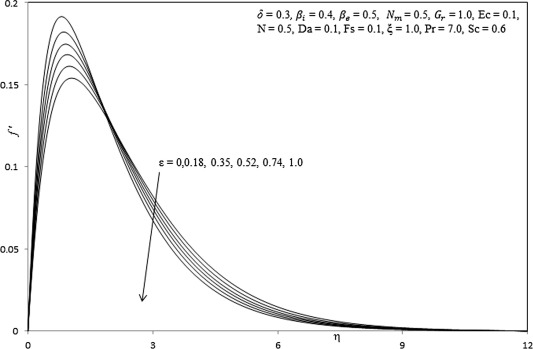Figure 2a. Influence of ${\textstyle \epsilon }$ on primary velocity profiles.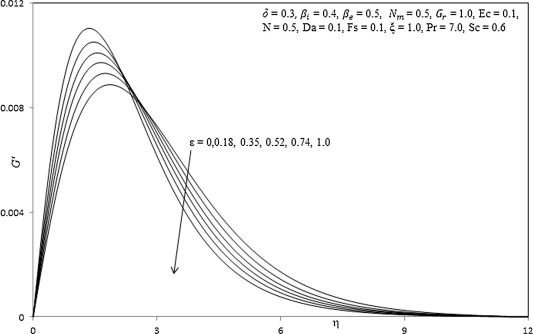Figure 2b. Influence of ${\textstyle \epsilon }$ on secondary velocity profiles.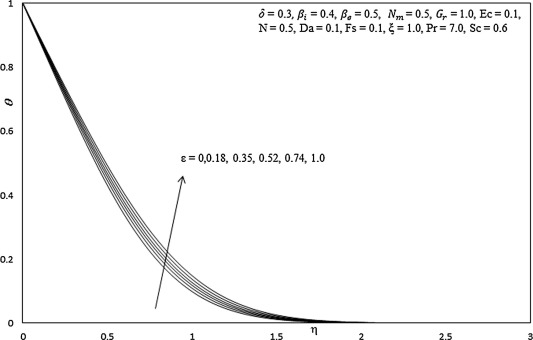Figure 2c. Influence of ${\textstyle \epsilon }$ on temperature profiles.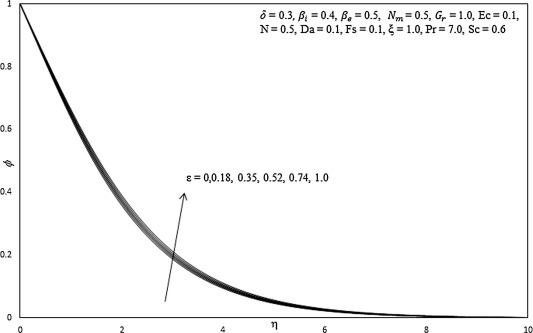Figure 2d. Influence of ${\textstyle \epsilon }$ on concentration profiles.

The thermal boundary layer Eq. (10) and the mass conservation Eq. (11a) and (11b) remain unchanged.

Figure 3a, Figure 3b, Figure 3c and Figure 3d illustrate the effect of the increasing local non-Newtonian fluid parameter, δ, primary velocity (f′), secondary velocity (G), temperature (θ) and concentration (ϕ) distributions through the boundary layer regime. Very little tangible effect is observed in Fig. 3a, although there is a very slight increase in primary and secondary velocity with increase in δ. Conversely, temperature and concentration are consistently reduced with increasing values of δ.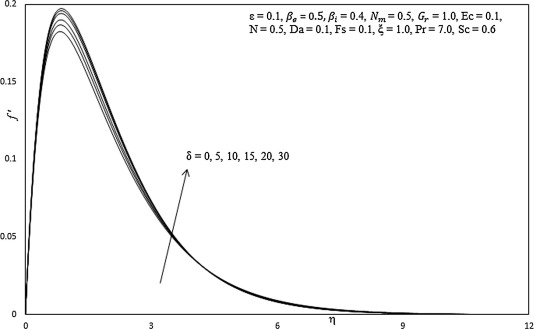Figure 3a. Influence of ${\textstyle \delta }$ on primary velocity profiles.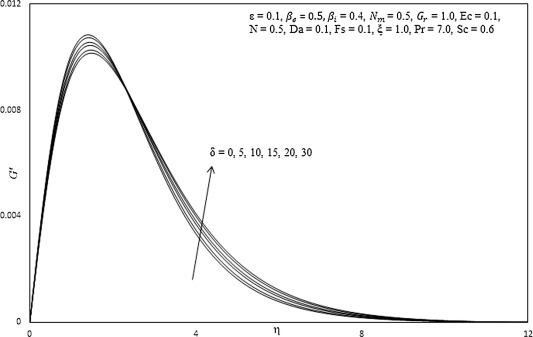Figure 3b. Influence of ${\textstyle \delta }$ on secondary velocity profiles.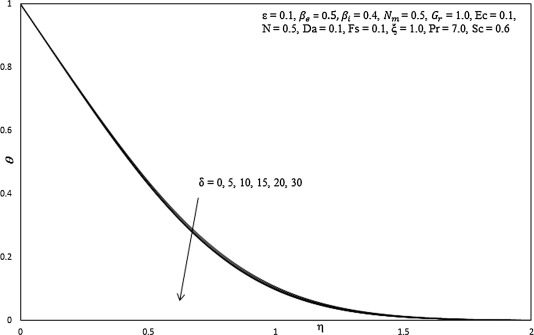Figure 3c. Influence of ${\textstyle \delta }$ on temperature profiles.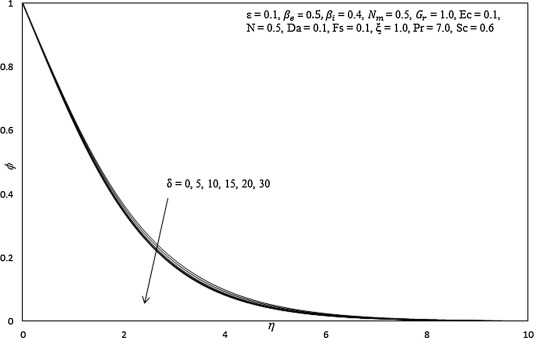Figure 3d. Influence of ${\textstyle \delta }$ on concentration profiles.

Figure 4a, Figure 4b, Figure 4c and Figure 4d, depict the evolution of primary velocity (f′), secondary velocity (G), temperature (θ) and concentration (ϕ) functions with a variation in Hall current parameter, βe. This parameter generates the cross flow effect in Magnetohydrodynamics. The Hall current parameter arises in both the primary and secondary momentum Eqs. (13) and (14) via the cross-flow   coupling terms, ${\textstyle -{\frac {2N_{m}}{\left[{\alpha }_{e}^{2}+{\beta }_{e}^{2}\right]}}\xi [{\alpha }_{e}f^{'}+}$${\beta }_{e}G]$ and ${\textstyle -{\frac {2N_{m}}{\left[{\alpha }_{e}^{2}+{\beta }_{e}^{2}\right]}}\xi [{\alpha }_{e}G-}$${\beta }_{e}f^{'}]$. It arises in quadratic form as a denominator i.e. ${\textstyle -{\frac {2N_{m}}{\left[{\alpha }_{e}^{2}+{\beta }_{e}^{2}\right]}}}$ in both and is coupled to the secondary velocity, G  , in the former, and to the primary velocity, ${\textstyle f^{'}}$ in the latter. The effect is for acceleration in the primary flow with greater Hall current parameter, as observed in Fig. 4a. The peak velocity is attained some distance from the plate surface. The primary velocity profiles in all cases converge asymptotically to vanishing free stream velocity, demonstrating that a sufficiently large infinity is prescribed in the computations. Momentum supplied to accelerate the primary flow is depleted from the secondary flow and this manifests in a strong deceleration in secondary velocity, as shown in Fig. 4b. With increasing Hall parameter, the peak secondary velocity is displaced closer to the plate surface. This does not occur for the primary velocity. A weak reduction in temperatures accompanies an increase in Hall parameter (Fig. 4c), which is attributable to the inverse square effect arising in the Joule heating term in Eq. (15), viz ${\textstyle +{\frac {2N_{m}Ec}{\left[{\alpha }_{e}^{2}+{\beta }_{e}^{2}\right]}}{\xi }^{3}[f^{{'}2}+}$$G^{2}]$ – this term couples the primary and secondary velocity fields to the energy field. For non-zero Eckert number, this term has a non-trivial effect on the temperature distribution. With greater Hall parameter the thermal boundary layer is cooled and thickness is decreased. Also a decrease in concentration is observed in Fig. 4d for increasing values of Hall parameter.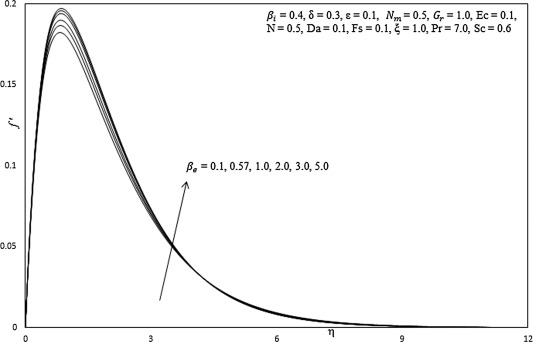Figure 4a. Influence of ${\textstyle {\beta }_{e}}$ on primary velocity profiles.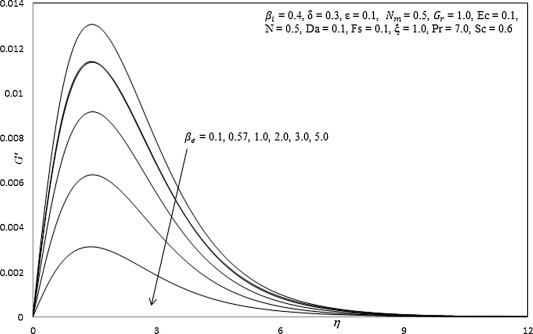Figure 4b. Influence of ${\textstyle {\beta }_{e}}$ on secondary velocity profiles.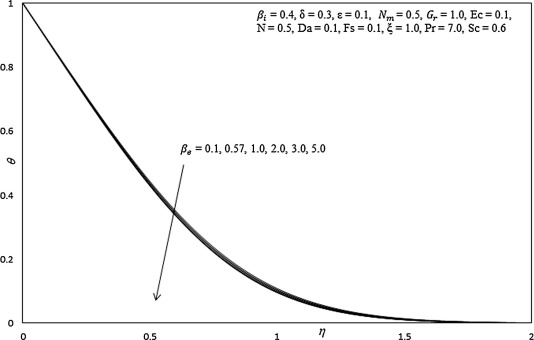Figure 4c. Influence of ${\textstyle {\beta }_{e}}$ on temperature profiles.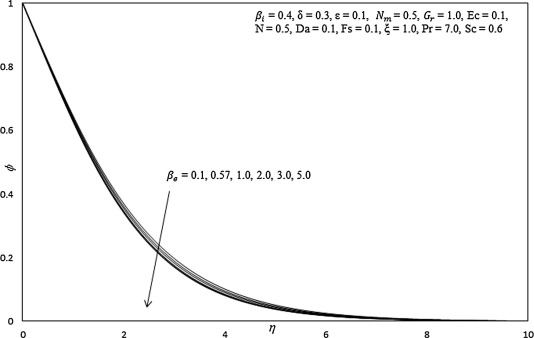Figure 4d. Influence of ${\textstyle {\beta }_{e}}$ on concentration profiles.

Figure 5a, Figure 5b, Figure 5c and Figure 5d illustrate the effect of the ionslip parameter i.e. βi on the primary velocity (f′), secondary velocity (G), temperature (θ) and concentration (ϕ) distributions through the boundary layer regime. Primary velocity is enhanced with increasing βi ( Fig. 5a). Conversely, secondary velocity, temperature and concentration are depressed with increasing βi values ( Figure 5b, Figure 5c and Figure 5d respectively). Ionslip arises in the parameter, αe = 1 + βiβe, which causes a heating effect as the ions slip in the magnetic field and a deceleration in the secondary flow. Only primary flow is positively influenced by the ionslip effect, and this characteristic is beneficial in MHD energy generator systems. It is also noteworthy to mention that generally values of primary velocity are several orders of magnitude greater than secondary velocity, a familiar phenomenon in MHD flows. Similar effects have been reported by other authors, although in the absence of non-isothermal plate conditions.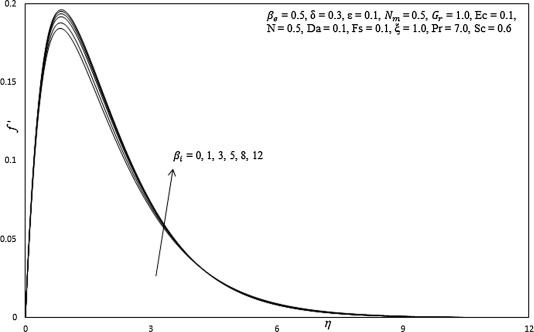Figure 5a. Influence of ${\textstyle {\beta }_{i}}$ on primary velocity profiles.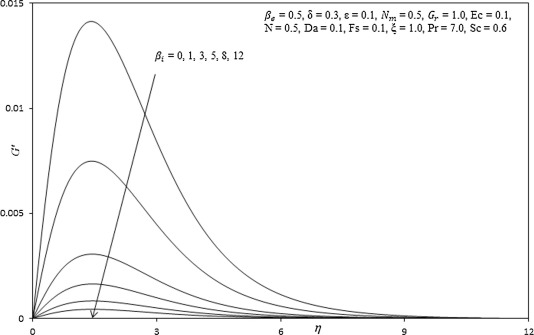Figure 5b. Influence of ${\textstyle {\beta }_{i}}$ on secondary velocity profiles.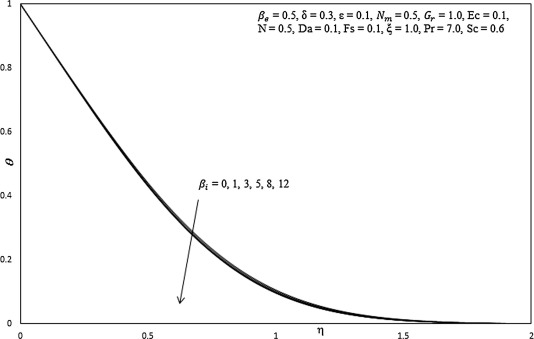Figure 5c. Influence of ${\textstyle {\beta }_{i}}$ on temperature profiles.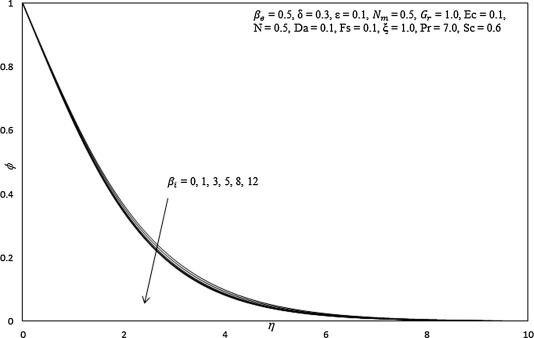Figure 5d. Influence of ${\textstyle {\beta }_{i}}$ on concentration profiles.

Figure 6a, Figure 6b, Figure 6c and Figure 6d present the distribution of primary velocity (f′), secondary velocity (G), temperature (θ) and concentration (ϕ  ) functions for various values of the magnetic body force parameter i.e. Hartmann number, ${\textstyle N_{m}={\frac {\sigma B_{o}^{2}L^{1/2}}{2\rho \nu C^{2}}}}$. An increase in Nm, strongly decelerates the flow i.e., reduces primary velocity values. In all profiles a peak arises near the surface of the plate and this peak is displaced progressively closer to the wall with an elevation in Nm values. This migration phenomenon has been reported by many other researchers . Essentially a greater retarding effect is generated in the flow with greater Nm values (i.e., stronger magnetic field strengths), which causes the prominent depression in velocities. For Nm = 1 the magnetic Lorentzian drag force will be of the same order of magnitude as the viscous hydrodynamic force. For Nm > 1, hydromagnetic drag will dominate and vice versa for Nm < 1. Therefore, in near-wall flows of magnetohydrodynamic generators or indeed materials processing, the flow can be very effectively controlled with a magnetic field. However, increasing Nm is found to accelerate the secondary velocity, temperature and concentration. The secondary flow acceleration is beneficial in certain manipulation processes in MHD materials technology as it encourages a more homogenous constitution in materials. The increase in temperature is generated by the dissipation in supplementary work expended in dragging the fluid against the action of the magnetic field. This work is converted to thermal energy which heats the fluid and enhances thermal boundary layer thickness.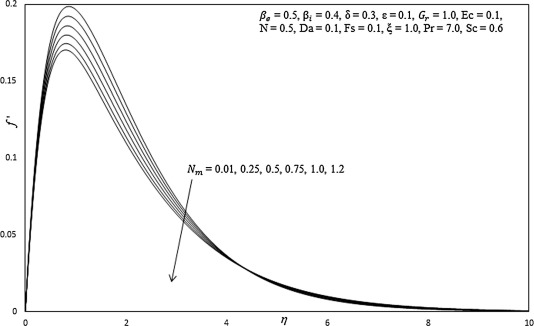Figure 6a. Influence of ${\textstyle N_{m}}$ on primary velocity profiles.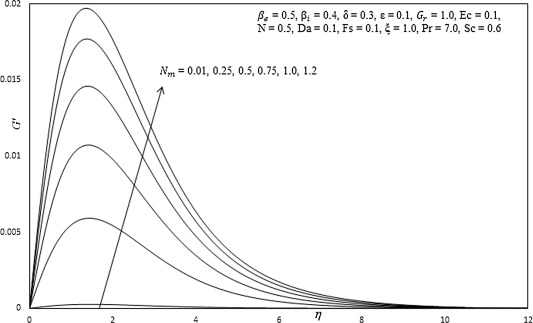Figure 6b. Influence of ${\textstyle N_{m}}$ on secondary velocity profiles.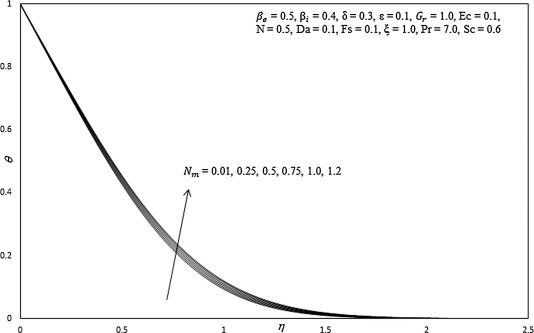Figure 6c. Influence of ${\textstyle N_{m}}$ on temperature profiles.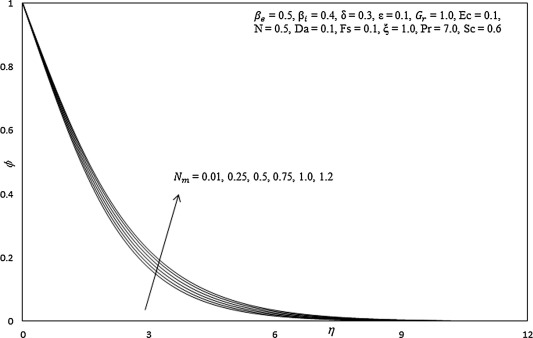Figure 6d. Influence of ${\textstyle N_{m}}$ on concentration profiles.

Figure 7a, Figure 7b, Figure 7c and Figure 7d depict the response of primary velocity (f′), secondary velocity (G), temperature (θ) and concentration (ϕ) to a variation in the Darcy parameter, Da. Primary and secondary velocities are clearly enhanced considerably with increasing Da as shown in Figure 7a and Figure 7b, since greater permeability of the regime corresponds to a decrease in Darcian drag force. The velocity peaks close to the plate surface are also found to be displaced further from the wall with increasing Darcy number. Porous media with greater permeability therefore assist both primary and secondary flow development whereas smaller permeability manifests in greater solid matrix impedance and decelerates both flow fields. A very strong decrease in temperature and concentration, as shown in Figure 7c and Figure 7d, occurs with increasing Da values. The progressive reduction in solid fibers in the porous medium with large Da values serves to decrease thermal conduction heat transfer in the regime. This inhibits the diffusion of thermal energy from the plate surface to the regime and cools the boundary layer also decreasing thermal boundary layer thickness. The presence of a porous medium therefore induces a significant effect on momentum and thermal diffusion in the system and provides a good mechanism for thermal regulation and flow control.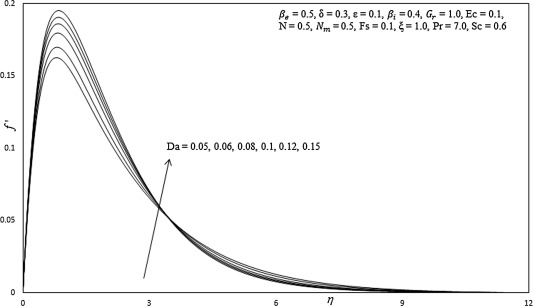Figure 7a. Influence of Da on primary velocity profiles.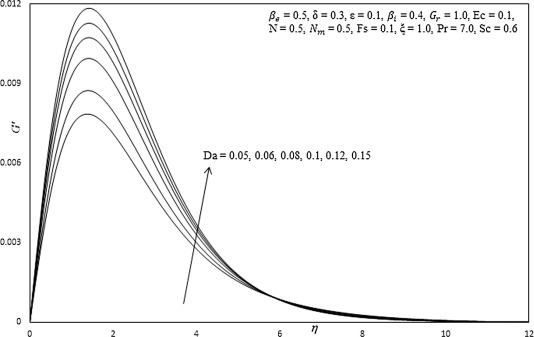Figure 7b. Influence of Da on secondary velocity profiles.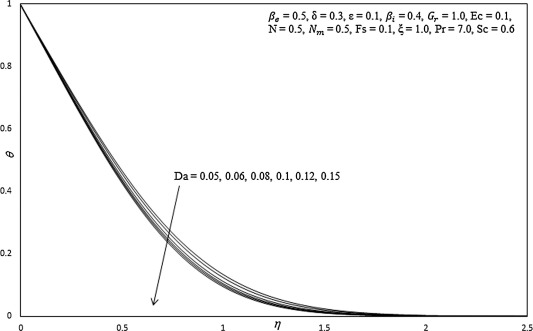Figure 7c. Influence of Da on temperature profiles.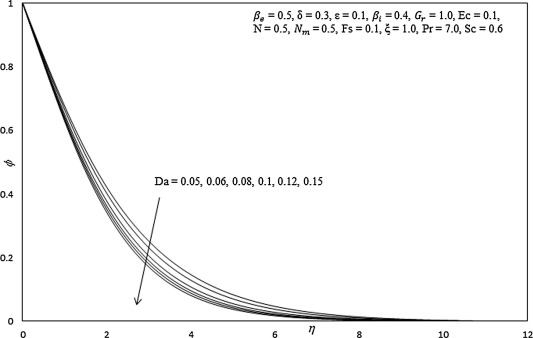Figure 7d. Influence of Da on concentration profiles.

Figure 8a, Figure 8b, Figure 8c and Figure 8d present the typical profiles for velocity functions, temperature and concentration distribution for various values of Forchheimer parameter, Fs. An increase in Fs markedly decelerates the flow as illustrated in Figure 8a and Figure 8b, for some considerable distance in the boundary layer, transverse to the plate surface. Inertial quadratic drag has a stronger effect closer to the wall. Forchheimer drag however is second order and the increase in this “form” drag effectively swamps the momentum development, thereby decelerating the flow, in particular near the plate surface. The term “non-Darcian” does not allude to a different regime of flow, but to the amplified effects of Forchheimer drag at higher velocities, as elaborated also in Anwar Bég et al.  and Prasad et al. . With a significant increase in Fs there is also a slight elevation in temperatures and concentrations ( Figure 8c and Figure 8d) in the regime. Thermal boundary layer thickness is therefore weakly enhanced with greater Forchheimer effect.Figure 8a. Influence of ${\textstyle Fs}$ on primary velocity profiles.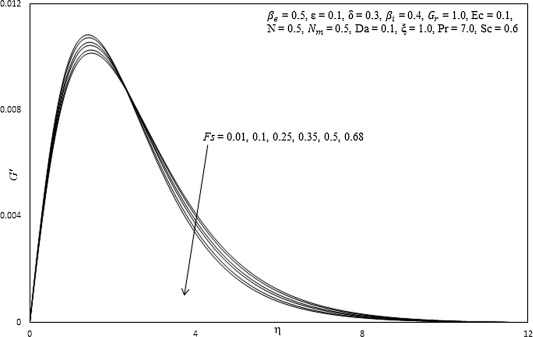Figure 8b. Influence of ${\textstyle Fs}$ on secondary velocity profiles.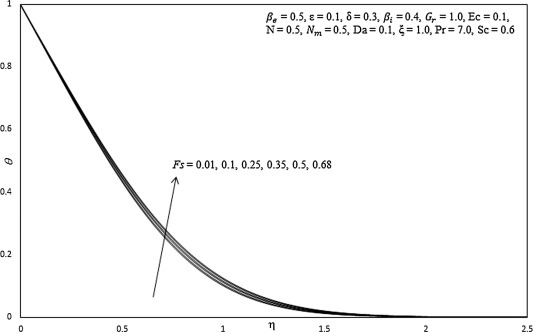Figure 8c. Influence of ${\textstyle Fs}$ on temperature profiles.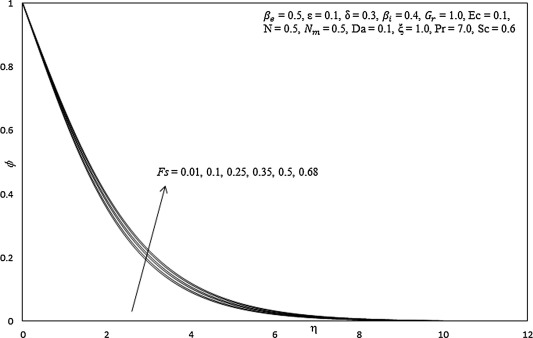Figure 8d. Influence of ${\textstyle Fs}$ on concentration profiles.

Figure 9a, Figure 9b and Figure 9c show the influence of Eyring–Powell fluid parameter, ε on dimensionless skin friction coefficient, heat and mass transfer rates. It is observed that the dimensionless skin friction is increased with the increase in ε i.e. the boundary layer flow is accelerated with decreasing viscosity effects in the non-Newtonian regime. Conversely, the surface heat and mass transfer rates are substantially decreased with increasing ε values. Decreasing viscosity of the fluid (induced by increasing the ε value) reduces thermal diffusion as compared with momentum diffusion.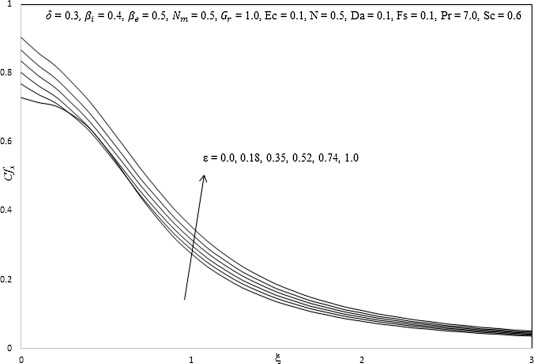Figure 9a. Influence of ${\textstyle \epsilon }$ on skin friction coefficient.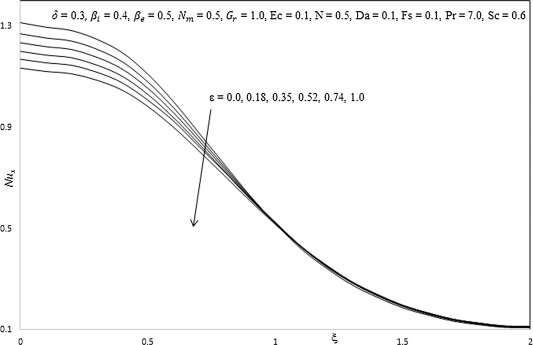Figure 9b. Influence of ${\textstyle \epsilon }$ on Nusselt number.Figure 9c. Influence of ${\textstyle \epsilon }$ on Sherwood number.

Figure 10a, Figure 10b and Figure 10c illustrate the influence of the local non-Newtonian parameter, δ, on the dimensionless skin friction coefficient, heat and mass transfer rate. The skin friction ( Fig. 10a) is accentuated with increasing δ, however only for very large values of the transverse coordinate, ξ. The flow is therefore strongly accelerated along the vertical surface far from the lower stagnation point. Heat and mass transfer rates are also enhanced with increasing δ, again at large values of ξ, as computed in Figure 10b and Figure 10c.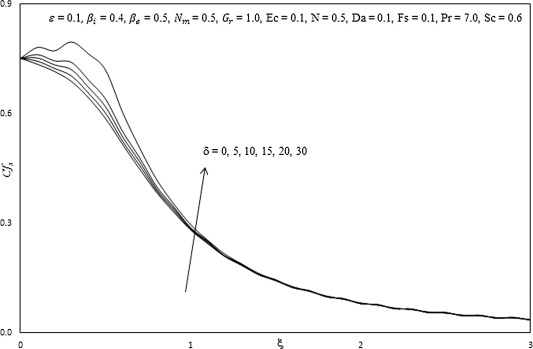Figure 10a. Influence of ${\textstyle \delta }$ on skin friction coefficient.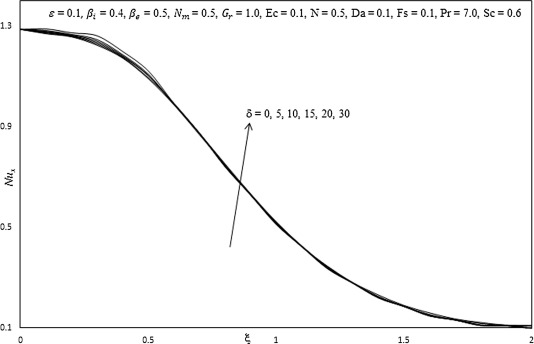Figure 10b. Influence of ${\textstyle \delta }$ on Nusselt number.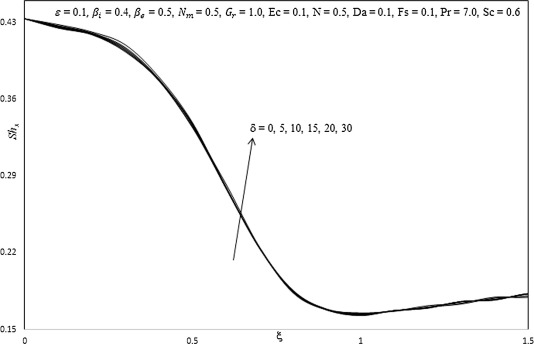Figure 10c. Influence of ${\textstyle \delta }$ on Sherwood number.

Figure 11a, Figure 11b and Figure 11c present the influence of increasing hall current parameter (βe) on the dimensionless skin friction coefficient, heat and mass transfer rate, along with a variation in transverse coordinate (ξ). With an increase in βe, the skin friction, heat and mass transfer rate are also found to increase.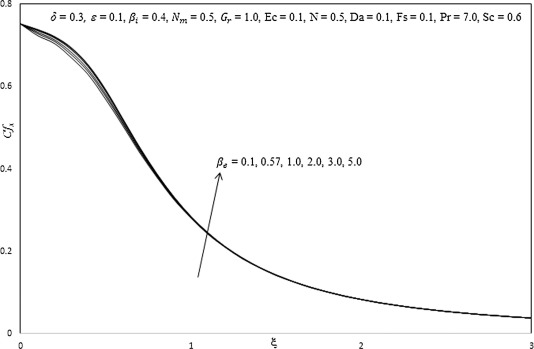Figure 11a. Influence of ${\textstyle {\beta }_{e}}$ on skin friction coefficient.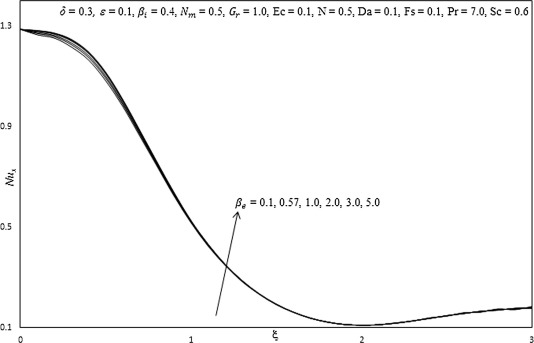Figure 11b. Influence of ${\textstyle {\beta }_{e}}$ on Nusselt number.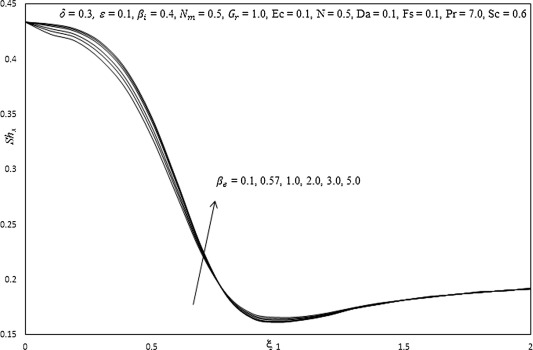Figure 11c. Influence of ${\textstyle {\beta }_{e}}$ on Sherwood number.

Figure 12a, Figure 12b and Figure 12c show the influence of increasing ionslip parameter (βi) on the dimensionless skin friction coefficient, heat and mass transfer rate along with a variation in transverse coordinate (ξ). With the increasing βi, a very slight increase is observed in the dimensionless skin friction coefficient, heat and mass transfer rate.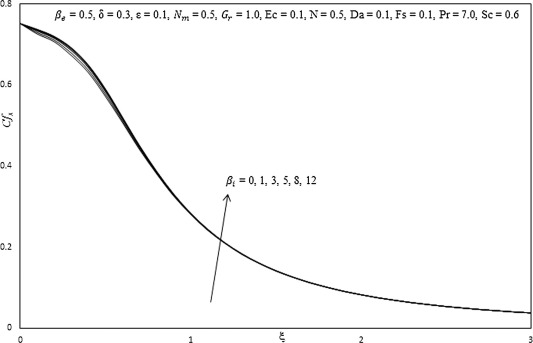Figure 12a. Influence of ${\textstyle {\beta }_{i}}$ on skin friction coefficient.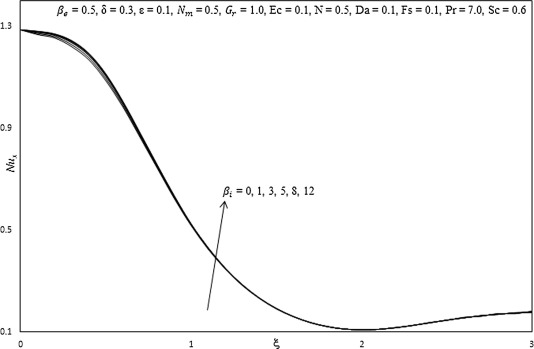Figure 12b. Influence of ${\textstyle {\beta }_{i}}$ on Nusselt number.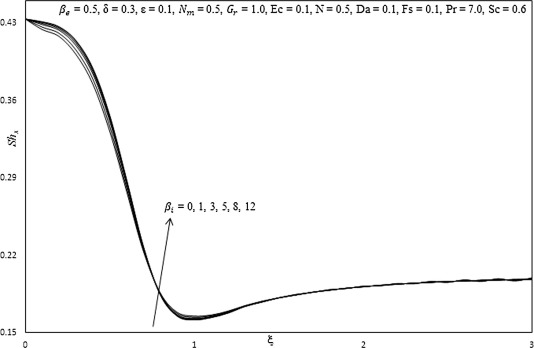Figure 12c. Influence of ${\textstyle {\beta }_{i}}$ on Sherwood number.

Figure 13a, Figure 13b and Figure 13c present the influence of increasing Hartmann (magnetic body force) number (Nm) on the dimensionless skin friction coefficient, heat and mass transfer rate, along with a variation in transverse coordinate (ξ). It is observed that increasing Nm, decreases the dimensionless skin friction coefficient, heat and mass transfer rate. This verifies earlier computations described showing that the flow is retarded with magnetic field but the fluid is heated. The transport of heat from the fluid to the plate corresponds to greater heat transfer rates and a drop in fluid temperature.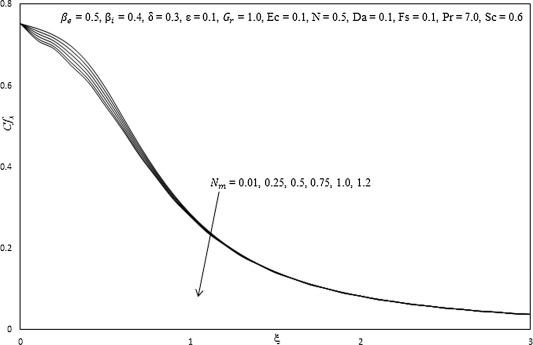Figure 13a. Influence of ${\textstyle N_{m}}$ on skin friction coefficient.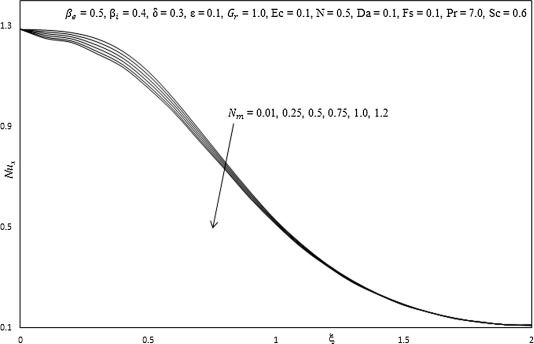Figure 13b. Influence of ${\textstyle N_{m}}$ on Nusselt number.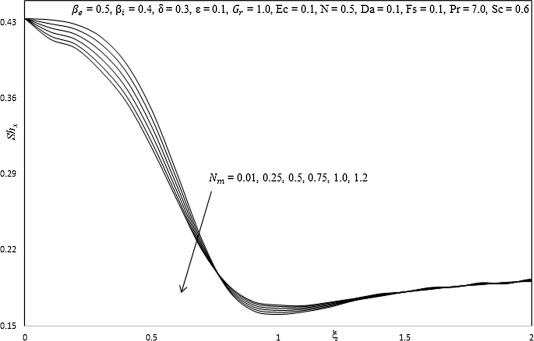Figure 13c. Influence of ${\textstyle N_{m}}$ on Sherwood number.

Figure 14a, Figure 14b and Figure 14c document the effect of increasing Darcy number (Da) on the dimensionless skin friction coefficient, heat and mass transfer rate, along with a variation in transverse coordinate (ξ). Increasing Da, is found to elevate the dimensionless skin friction coefficient, heat and mass transfer rate.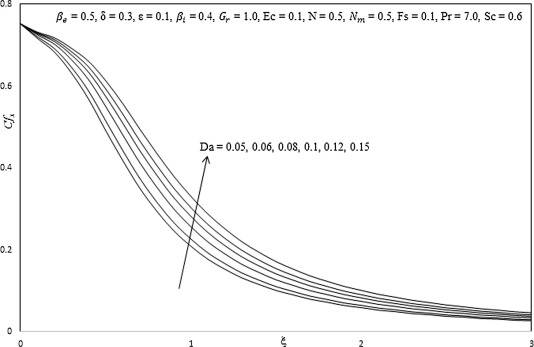Figure 14a. Influence of Da on skin friction coefficient.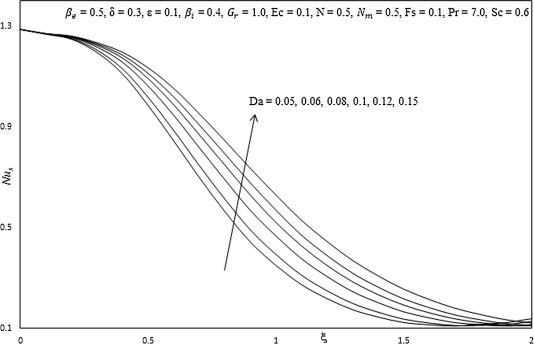Figure 14b. Influence of Da on Nusselt number.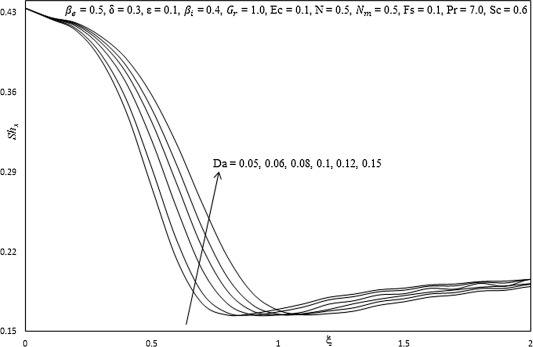Figure 14c. Influence of Da on Sherwood number.

Figure 15a, Figure 15b and Figure 15c document the effect of increasing Forchheimer quadratic drag number (Fs) on the dimensionless skin friction coefficient, heat and mass transfer rate, along with a variation in transverse coordinate (ξ). Increasing Fs, is found to decrease the dimensionless skin friction coefficient, heat and mass transfer rate.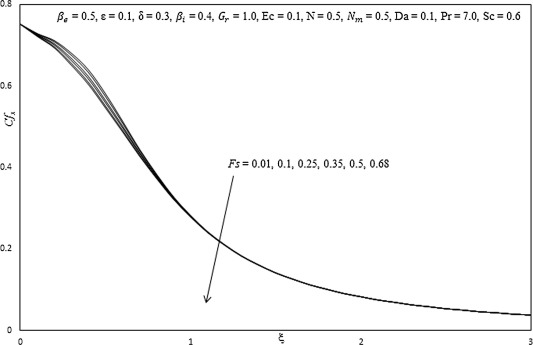Figure 15a. Influence of ${\textstyle Fs}$ on skin friction coefficient.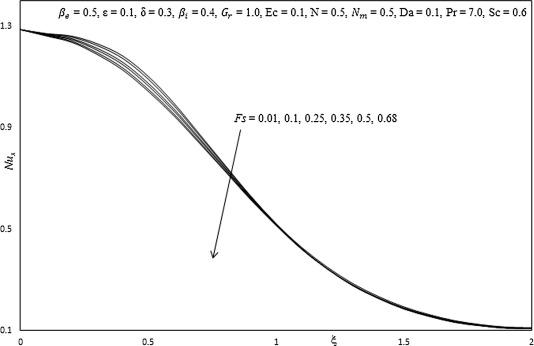Figure 15b. Influence of ${\textstyle Fs}$ on Nusselt number.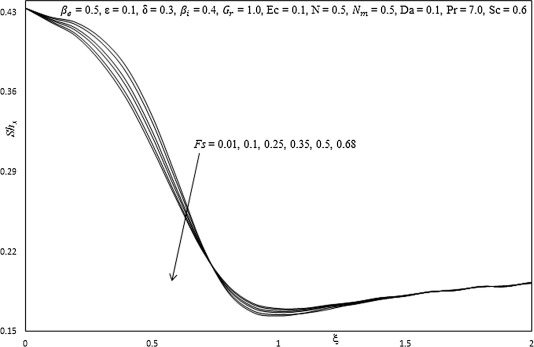Figure 15c. Influence of ${\textstyle Fs}$ on Sherwood number.

Table 1 presents the influence of increasing Eyring–Powell fluid parameter (ε), Hall current parameter (βe) and ionslip parameter (βi) on skin friction coefficient, heat transfer rate and mass transfer rate, along with a variation in transverse coordinate (ξ). Increasing ε, increases the skin friction coefficient, whereas, decreases heat transfer rate and mass transfer rate. An increasing βe, is found to increase skin friction coefficient, heat transfer rate and mass transfer rate. Increasing βi, is observed to increase the skin friction coefficient, heat transfer rate and mass transfer rate.

Table 2 presents the influence of increasing Schmidt number (Sc) and Prandtl number (Pr) on skin friction coefficient, heat transfer rate and mass transfer rate along with a variation in transverse coordinate (ξ). It is observed that the skin friction coefficient is decreased slightly with increasing Sc. The heat transfer rate is reduced with increasing values of Sc but mass transfer rate is enhanced with increasing Sc. Increasing Pr is found to decrease the skin friction coefficient and mass transfer rate whereas the heat transfer rate is enhanced.

Table 3 documents the effect of increasing local non-Newtonian parameter based on length scale (δ), Forchheimer quadratic drag number (Fs) and Hartmann (magnetic body force) number (Nm) on skin friction coefficient, heat transfer rate and mass transfer rate, along with a variation in transverse coordinate (ξ). Increasing δ is found to increase skin friction coefficient, heat transfer rate and mass transfer rate. Conversely, increasing Nm decreases skin friction coefficient, heat transfer rate and mass transfer rate. The transport of heat from the fluid to the plate corresponds to greater heat transfer rates and a drop in fluid temperature. Also, increasing Fs is observed to decrease skin friction coefficient, heat transfer rate and mass transfer rate Fs.

Table 4 presents the influence of increasing Darcy parameter (Da) and Buoyancy ratio parameter (N) on skin friction coefficient, heat transfer rate and mass transfer rate, along with a variation in transverse coordinate (ξ). It is observed that the skin friction coefficient, heat transfer rate and mass transfer rate are enhanced with increasing Da. It is also observed that increasing N increases the skin friction coefficient and heat transfer rate but decreases the mass transfer rate.

## 9. Conclusions

A mathematical model has been developed for the steady hydromagnetic free convection heat and mass transfer of non-Newtonian Eyring–Powell electrically-conducting fluid from a non-isothermal vertical surface adjacent to a non-Darcian porous medium in the presence of Hall currents, ionslip currents and viscous and Joule heating effects. The boundary value problem (BVP) has been transformed from an (xyz) coordinate system to a (ξη) coordinate system. A number of important special cases have been described in addition to key engineering parameters being derived. The Keller box method has been employed to obtain implicit finite difference numerical solutions for the solution of the strongly coupled, nonlinear two-point boundary value problem. Extensive computations have been presented for the effects of Eyring–Powell fluid parameter (ε), local non-Newtonian parameter based on length scale x (δ), Darcy number (Da), Forchheimer number (Fs), hydromagnetic parameter (Nm), Hall current parameter (βe), ionslip parameter (βi), on the velocity fields and temperature distribution in the regime, with prescribed Eckert number (Ec), Schmidt number (Sc) and Prandtl number (Pr). Computations have been validated with Nakamura’s tridiagonal difference method (NTM). A strong deceleration in primary and secondary flow has been shown to arise with greater non-isothermal effect at the wall, also leading to a decrease in temperatures. Increasing Hall parameter and ionslip are observed to accelerate weakly the primary flow but to strongly retard the secondary flow. Increasing Darcy number accelerates both primary and secondary flow but cools the fluid, as does an increase in Forchheimer number. The current study finds applications in both magnetic materials fabrication (e.g. liquid metal flows) and MHD energy generator near-wall flows. It also renders some useful benchmarks for more generalized commercial CFD simulations.

## Acknowledgments

The authors are grateful to both reviewers and their comments which served to significantly improve the interpretative and other aspects of the present article.

### Document informationPublished on 12/04/17

Licence: Other

### Document Score0

Views 94
Recommendations 0